# ModelViewController Architecture CS 5010 Program Design Paradigms Bootcamp

• Slides: 29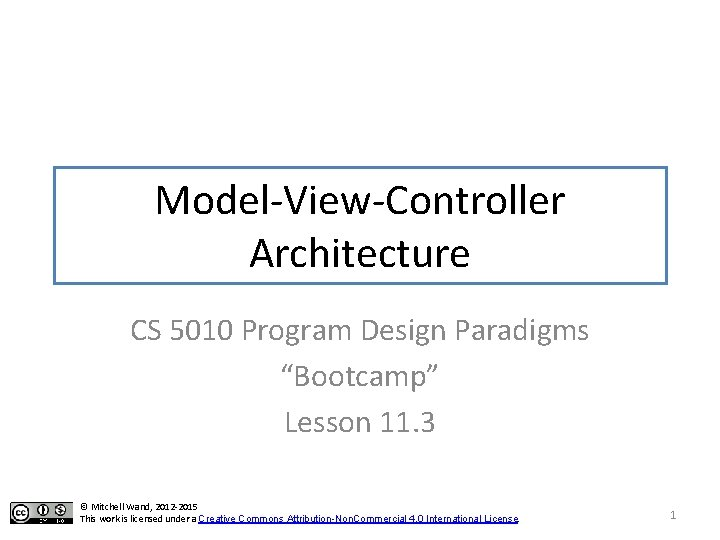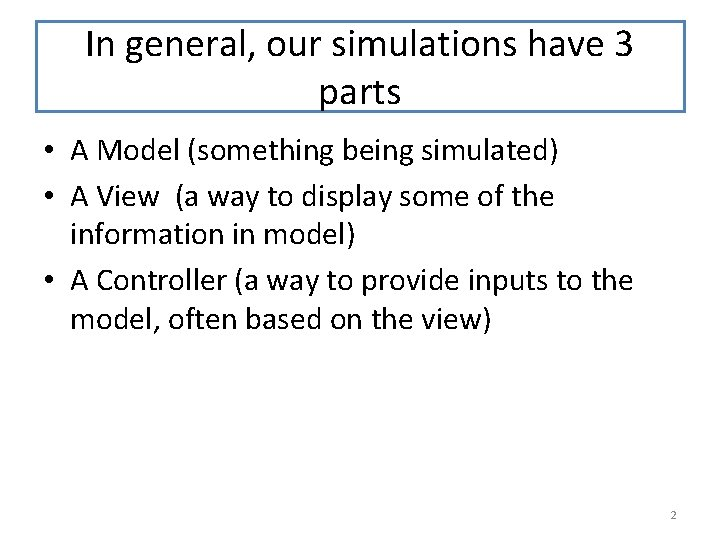In general, our simulations have 3 parts • A Model (something being simulated) • A View (a way to display some of the information in model) • A Controller (a way to provide inputs to the model, often based on the view) 2Helpful to separate these • Each part may be complicated (separation of concerns) • Model shouldn't care about how it is displayed • May have several viewers and controllers • Model and viewer may be running at different rates • Clarify interface between controller and model. 3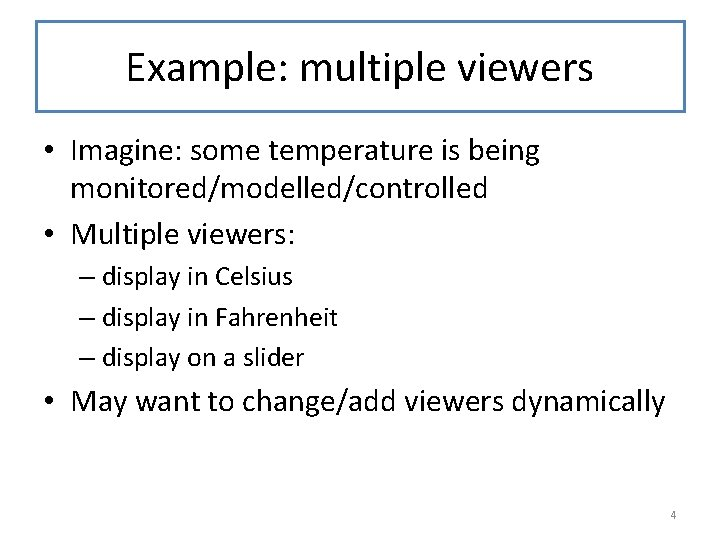Example: multiple viewers • Imagine: some temperature is being monitored/modelled/controlled • Multiple viewers: – display in Celsius – display in Fahrenheit – display on a slider • May want to change/add viewers dynamically 4Larger Example: Nuclear Reactor • The reactor has many valves, sensors, etc. • Unlike our screensavers, balls, etc. there’s no single object to display. • Best we can do is to have a viewer for each sensor and a controller for each valve. • People add new sensors and new valves all the time. • How can we model this? 5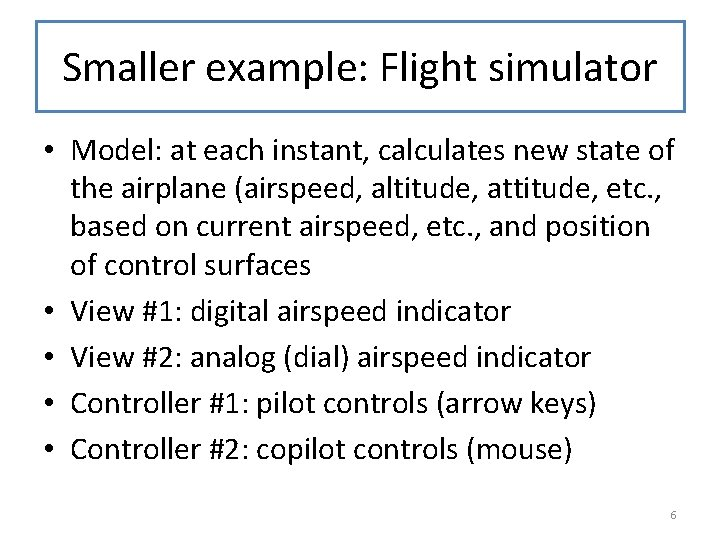Smaller example: Flight simulator • Model: at each instant, calculates new state of the airplane (airspeed, altitude, attitude, etc. , based on current airspeed, etc. , and position of control surfaces • View #1: digital airspeed indicator • View #2: analog (dial) airspeed indicator • Controller #1: pilot controls (arrow keys) • Controller #2: copilot controls (mouse) 6Our current architecture has these all mixed together • Every Widget<%> or SWidget<%> is responsible for all 3 aspects: – on-tick (Model) – add-to-scene (View) – on-mouse, on-key (Controller). • What can we do about this? 7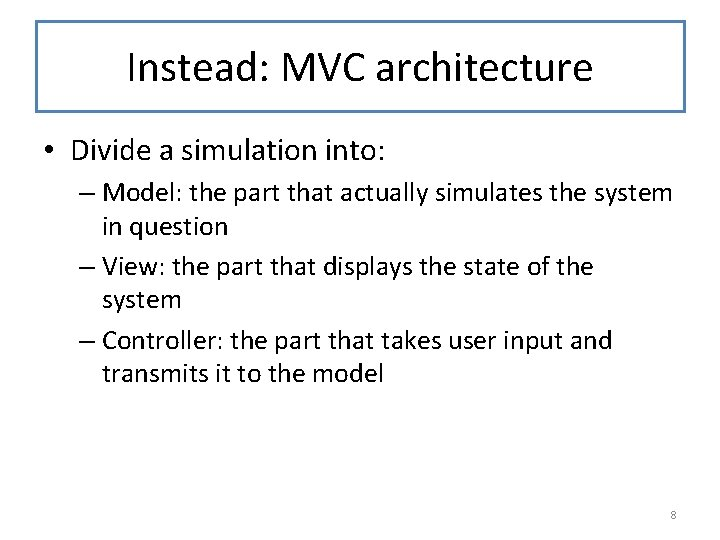Instead: MVC architecture • Divide a simulation into: – Model: the part that actually simulates the system in question – View: the part that displays the state of the system – Controller: the part that takes user input and transmits it to the model 8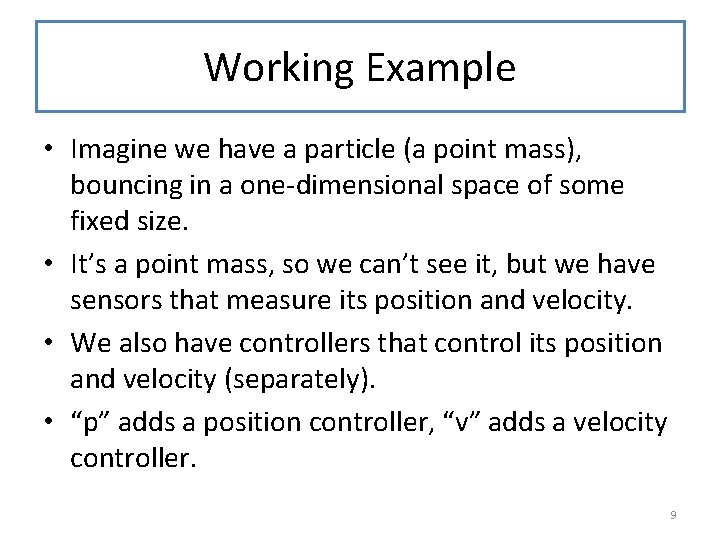Working Example • Imagine we have a particle (a point mass), bouncing in a one-dimensional space of some fixed size. • It’s a point mass, so we can’t see it, but we have sensors that measure its position and velocity. • We also have controllers that control its position and velocity (separately). • “p” adds a position controller, “v” adds a velocity controller. 9Demonstration 10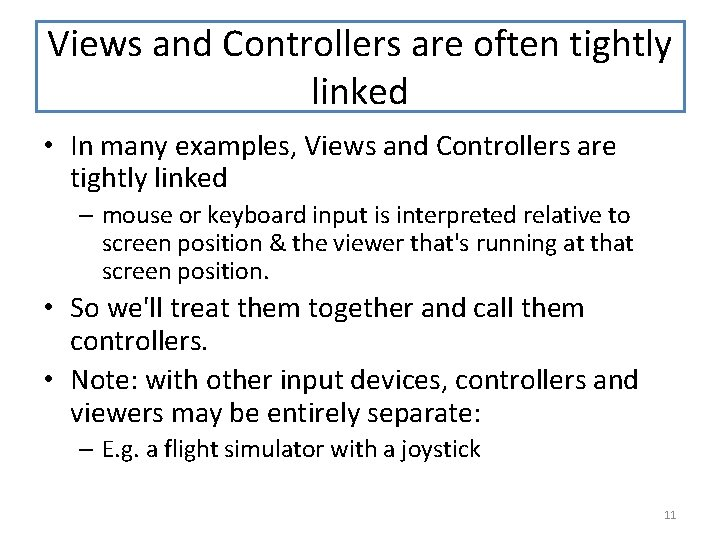Views and Controllers are often tightly linked • In many examples, Views and Controllers are tightly linked – mouse or keyboard input is interpreted relative to screen position & the viewer that's running at that screen position. • So we'll treat them together and call them controllers. • Note: with other input devices, controllers and viewers may be entirely separate: – E. g. a flight simulator with a joystick 11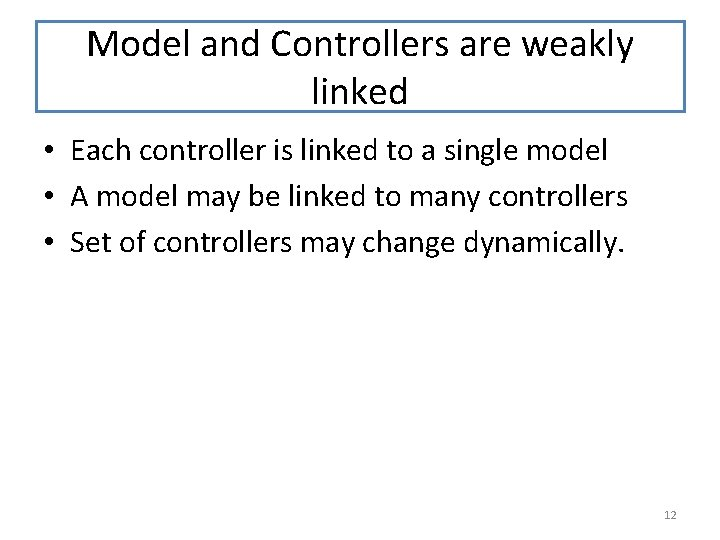Model and Controllers are weakly linked • Each controller is linked to a single model • A model may be linked to many controllers • Set of controllers may change dynamically. 12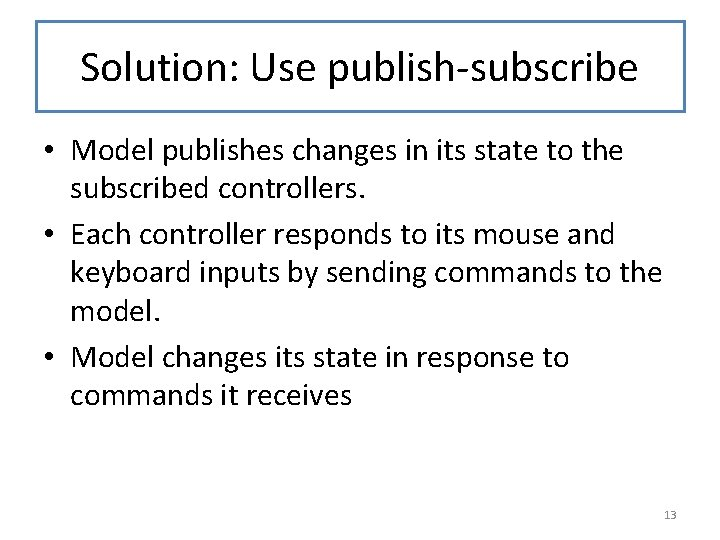Solution: Use publish-subscribe • Model publishes changes in its state to the subscribed controllers. • Each controller responds to its mouse and keyboard inputs by sending commands to the model. • Model changes its state in response to commands it receives 13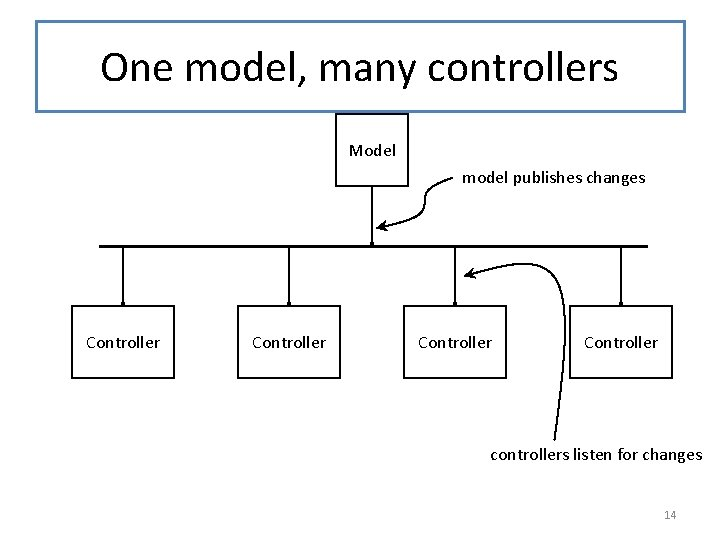One model, many controllers Model model publishes changes Controller controllers listen for changes 14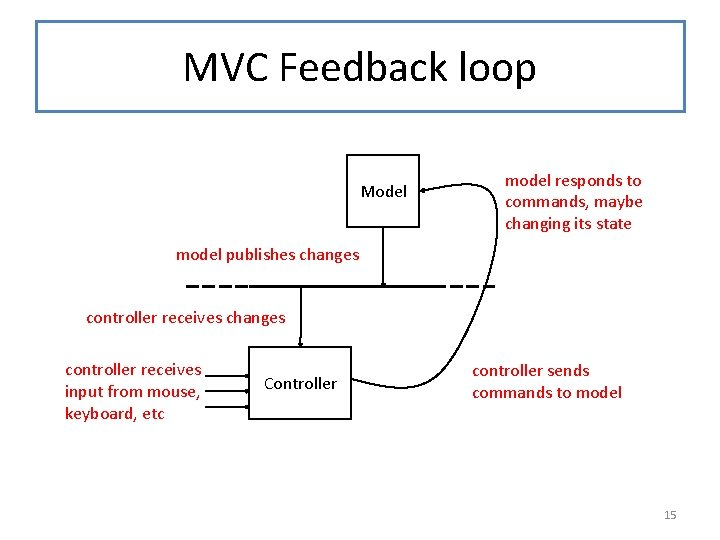MVC Feedback loop Model model responds to commands, maybe changing its state model publishes changes controller receives input from mouse, keyboard, etc Controller controller sends commands to model 15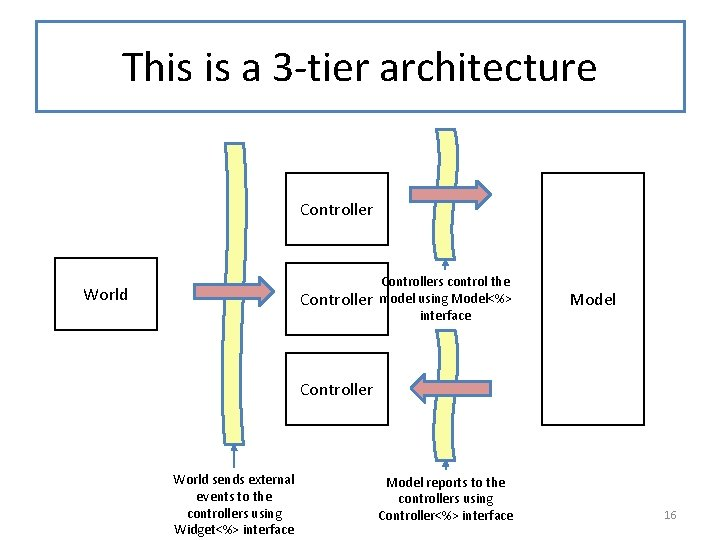This is a 3 -tier architecture Controller World Controllers control the model using Model<%> interface Model Controller World sends external events to the controllers using Widget<%> interface Model reports to the controllers using Controller<%> interface 16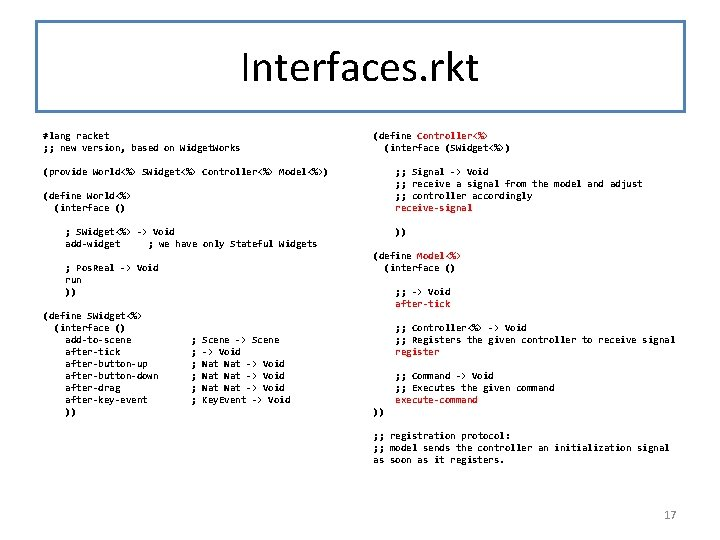Interfaces. rkt #lang racket ; ; new version, based on Widget. Works (define Controller<%> (interface (SWidget<%>) (provide World<%> SWidget<%> Controller<%> Model<%>) ; ; Signal -> Void ; ; receive a signal from the model and adjust ; ; controller accordingly receive-signal (define World<%> (interface () ; SWidget<%> -> Void add-widget ; we have only Stateful Widgets (define Model<%> (interface () ; Pos. Real -> Void run )) (define SWidget<%> (interface () add-to-scene after-tick after-button-up after-button-down after-drag after-key-event )) )) ; ; -> Void after-tick ; ; ; Controller<%> -> Void ; ; Registers the given controller to receive signal register Scene -> Void Nat Nat -> Void Key. Event -> Void ; ; Command -> Void ; ; Executes the given command execute-command )) ; ; registration protocol: ; ; model sends the controller an initialization signal as soon as it registers. 17Data Definitions for Communicating with Model (define-struct set-position-command (pos) #: transparent) (define-struct incr-velocity-command (dv) #: transparent) ; ; A Command is one of ; ; -- (make-set-position Real) ; ; INTERP: set the position of the particle to pos ; ; -- (make-incr-velocity Real) ; ; INTERP: increment the velocity of the particle by dv (define-struct position-signal (pos) #: transparent) (define-struct velocity-signal (v) #: transparent) ; ; A Signal is one of ; ; -- (make-position-signal Real) ; ; -- (make-velocity-signal Real) ; ; INTERP: report the current position or velocity of the ; ; particle 18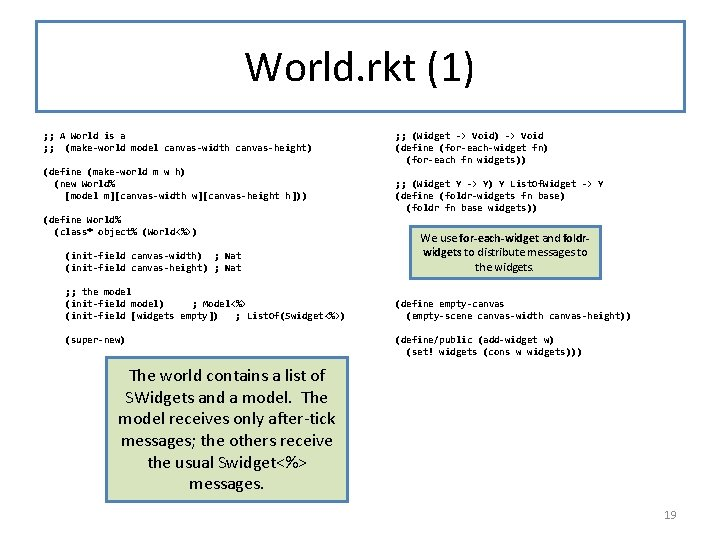World. rkt (1) ; ; A World is a ; ; (make-world model canvas-width canvas-height) (define (make-world m w h) (new World% [model m][canvas-width w][canvas-height h])) (define World% (class* object% (World<%>) (init-field canvas-width) ; Nat (init-field canvas-height) ; Nat ; ; the model (init-field model) ; Model<%> (init-field [widgets empty]) ; List. Of(Swidget<%>) (super-new) ; ; (Widget -> Void) -> Void (define (for-each-widget fn) (for-each fn widgets)) ; ; (Widget Y -> Y) Y List. Of. Widget -> Y (define (foldr-widgets fn base) (foldr fn base widgets)) We use for-each-widget and foldrwidgets to distribute messages to the widgets. (define empty-canvas (empty-scene canvas-width canvas-height)) (define/public (add-widget w) (set! widgets (cons w widgets))) The world contains a list of SWidgets and a model. The model receives only after-tick messages; the others receive the usual Swidget<%> messages. 19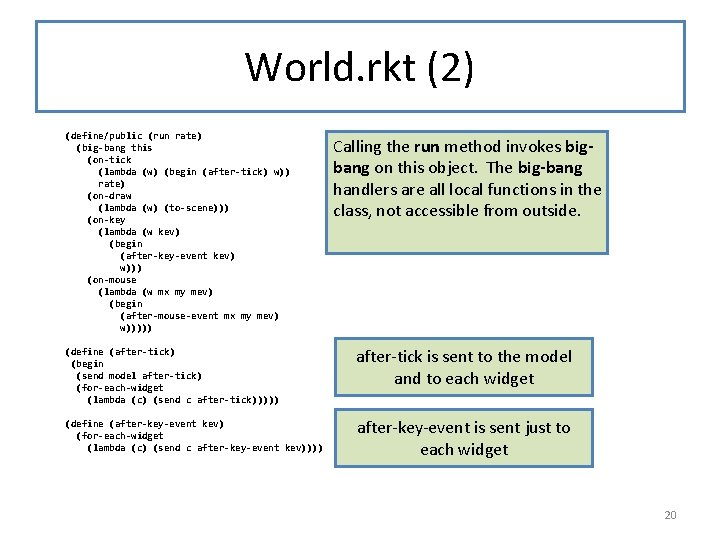World. rkt (2) (define/public (run rate) (big-bang this (on-tick (lambda (w) (begin (after-tick) w)) rate) (on-draw (lambda (w) (to-scene))) (on-key (lambda (w kev) (begin (after-key-event kev) w))) (on-mouse (lambda (w mx my mev) (begin (after-mouse-event mx my mev) w))))) Calling the run method invokes bigbang on this object. The big-bang handlers are all local functions in the class, not accessible from outside. (define (after-tick) (begin (send model after-tick) (for-each-widget (lambda (c) (send c after-tick))))) after-tick is sent to the model and to each widget (define (after-key-event kev) (for-each-widget (lambda (c) (send c after-key-event kev)))) after-key-event is sent just to each widget 20World. rkt (3) (define (to-scene) (foldr-widgets (lambda (widget scene) (send widget add-to-scene)) empty-canvas)) ; ; decode the mouse event and send ; ; button-down/drag/button-up ; ; events to each widget (define (after-mouse-event mx my mev) (for-each-widget (mouse-event->message mx my mev))) ; ; Nat Mouse. Event -> (Widget -> Void) (define (mouse-event->message mx my mev) (cond [(mouse=? mev "button-down") (lambda (obj) (send obj after-button-down mx my))] [(mouse=? mev "drag") (lambda (obj) (send obj after-drag mx my))] [(mouse=? mev "button-up") (lambda (obj) (send obj after-button-up mx my))] [else (lambda (obj) 1111)])) )) to-scene calls the add-toscene method on each widget, and folds the results after-mouse-event decodes the mouse event and sends the appropriate method call to each widget. This version of the code breaks up the task differently than Widget. Works. rkt did. Do you understand how each version works? Puzzle: why must the else line be of the form (lambda (obj). . ) ? 21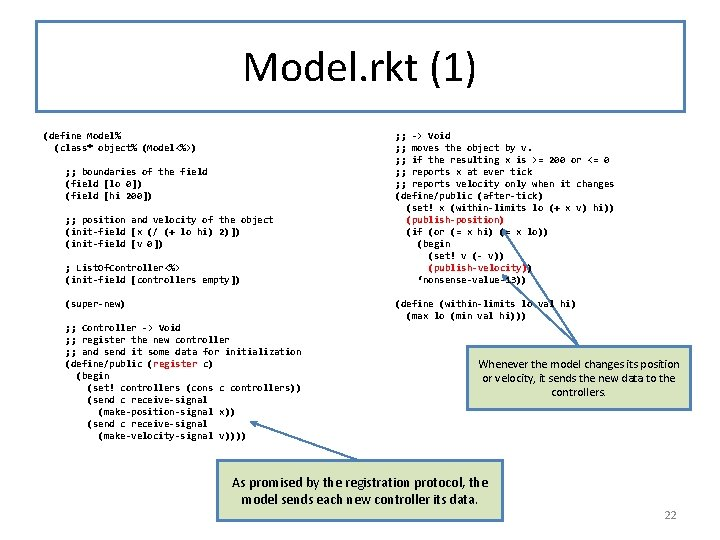Model. rkt (1) (define Model% (class* object% (Model<%>) ; ; boundaries of the field (field [lo 0]) (field [hi 200]) ; ; position and velocity of the object (init-field [x (/ (+ lo hi) 2)]) (init-field [v 0]) ; List. Of. Controller<%> (init-field [controllers empty]) (super-new) ; ; -> Void ; ; moves the object by v. ; ; if the resulting x is >= 200 or <= 0 ; ; reports x at ever tick ; ; reports velocity only when it changes (define/public (after-tick) (set! x (within-limits lo (+ x v) hi)) (publish-position) (if (or (= x hi) (= x lo)) (begin (set! v (- v)) (publish-velocity)) ‘nonsense-value-13)) (define (within-limits lo val hi) (max lo (min val hi))) ; ; Controller -> Void ; ; register the new controller ; ; and send it some data for initialization (define/public (register c) (begin (set! controllers (cons c controllers)) (send c receive-signal (make-position-signal x)) (send c receive-signal (make-velocity-signal v)))) Whenever the model changes its position or velocity, it sends the new data to the controllers. As promised by the registration protocol, the model sends each new controller its data. 22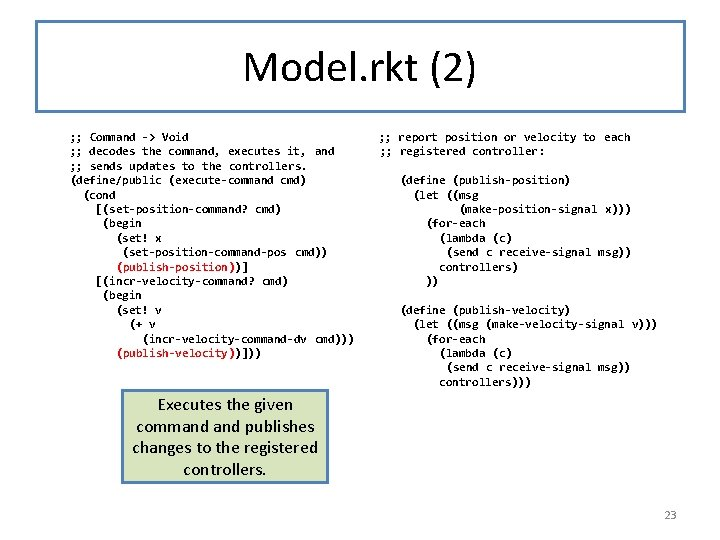Model. rkt (2) ; ; Command -> Void ; ; decodes the command, executes it, and ; ; sends updates to the controllers. (define/public (execute-command cmd) (cond [(set-position-command? cmd) (begin (set! x (set-position-command-pos cmd)) (publish-position))] [(incr-velocity-command? cmd) (begin (set! v (+ v (incr-velocity-command-dv cmd))) (publish-velocity))])) ; ; report position or velocity to each ; ; registered controller: (define (publish-position) (let ((msg (make-position-signal x))) (for-each (lambda (c) (send c receive-signal msg)) controllers) )) (define (publish-velocity) (let ((msg (make-velocity-signal v))) (for-each (lambda (c) (send c receive-signal msg)) controllers))) Executes the given command publishes changes to the registered controllers. 23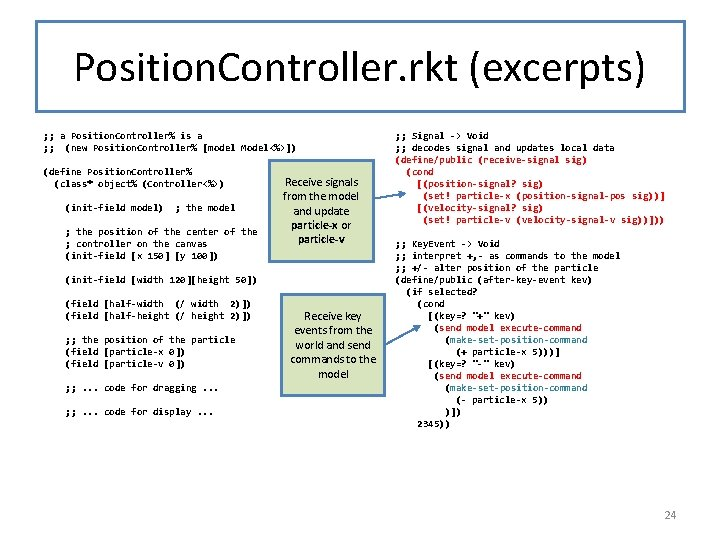Position. Controller. rkt (excerpts) ; ; a Position. Controller% is a ; ; (new Position. Controller% [model Model<%>]) (define Position. Controller% (class* object% (Controller<%>) (init-field model) ; the model ; the position of the center of the ; controller on the canvas (init-field [x 150] [y 100]) Receive signals from the model and update particle-x or particle-v (init-field [width 120][height 50]) (field [half-width (/ width 2)]) (field [half-height (/ height 2)]) ; ; the position of the particle (field [particle-x 0]) (field [particle-v 0]) ; ; . . . code for dragging. . . ; ; . . . code for display. . . Receive key events from the world and send commands to the model ; ; Signal -> Void ; ; decodes signal and updates local data (define/public (receive-signal sig) (cond [(position-signal? sig) (set! particle-x (position-signal-pos sig))] [(velocity-signal? sig) (set! particle-v (velocity-signal-v sig))])) ; ; Key. Event -> Void ; ; interpret +, - as commands to the model ; ; +/- alter position of the particle (define/public (after-key-event kev) (if selected? (cond [(key=? "+" kev) (send model execute-command (make-set-position-command (+ particle-x 5)))] [(key=? "-" kev) (send model execute-command (make-set-position-command (- particle-x 5)) )]) 2345)) 24Velocity. Controller. rkt (define Velocity. Controller% (class* object% (Controller<%>) (init-field model) ; the model ; the position of the center of the ; controller on the canvas (init-field [x 150] [y 100]) setup, signalreception just the same (init-field [width 120][height 50]) (field [half-width (/ width 2)]) (field [half-height (/ height 2)]) ; ; the position of the particle (field [particle-x 0]) (field [particle-v 0]) ; ; . . . code for dragging. . . ; ; . . . code for display. . . + and – are interpreted differently: as commands to change the velocity of the model. Notice that the commands form a rudimentary programming language. ; ; Signal -> Void ; ; decodes signal and updates local data (define/public (receive-signal sig) (cond [(report-position? sig) (set! particle-x (report-position-pos sig))] [(report-velocity? sig) (set! particle-v (report-velocity-v sig))])) ; ; Key. Event -> Void ; ; interpret +, - as commands to the model ; ; +/- alter velocity of the particle (define/public (after-key-event kev) (if selected? (cond [(key=? "+" kev) (send model execute-command (make-incr-velocity-command 1))] [(key=? "-" kev) (send model execute-command (make-incr-velocity-command -1))]) 3456)) Lots of opportunity here for sharing implementation via inheritance; we just haven’t done so. 25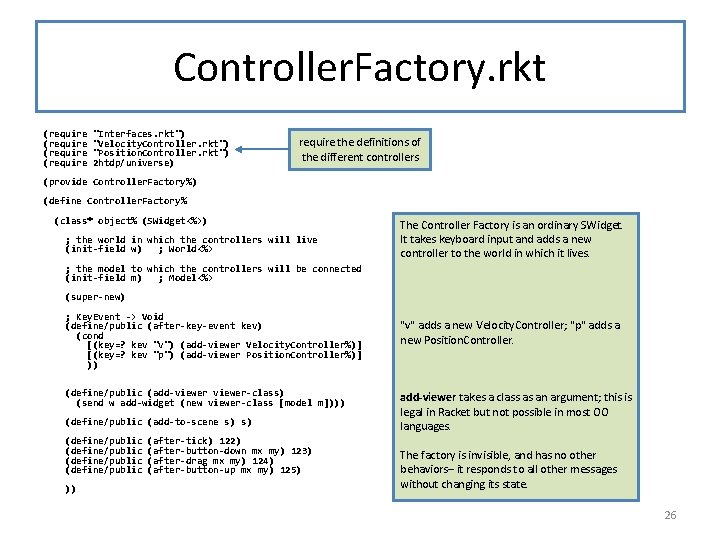Controller. Factory. rkt (require "Interfaces. rkt") "Velocity. Controller. rkt") "Position. Controller. rkt") 2 htdp/universe) require the definitions of the different controllers (provide Controller. Factory%) (define Controller. Factory% (class* object% (SWidget<%>) ; the world in which the controllers will live (init-field w) ; World<%> The Controller Factory is an ordinary SWidget. It takes keyboard input and adds a new controller to the world in which it lives. ; the model to which the controllers will be connected (init-field m) ; Model<%> (super-new) ; Key. Event -> Void (define/public (after-key-event kev) (cond [(key=? kev "v") (add-viewer Velocity. Controller%)] [(key=? kev "p") (add-viewer Position. Controller%)] )) (define/public (add-viewer-class) (send w add-widget (new viewer-class [model m]))) (define/public (add-to-scene s) s) (define/public )) (after-tick) 122) (after-button-down mx my) 123) (after-drag mx my) 124) (after-button-up mx my) 125) "v" adds a new Velocity. Controller; "p" adds a new Position. Controller. add-viewer takes a class as an argument; this is legal in Racket but not possible in most OO languages. The factory is invisible, and has no other behaviors– it responds to all other messages without changing its state. 26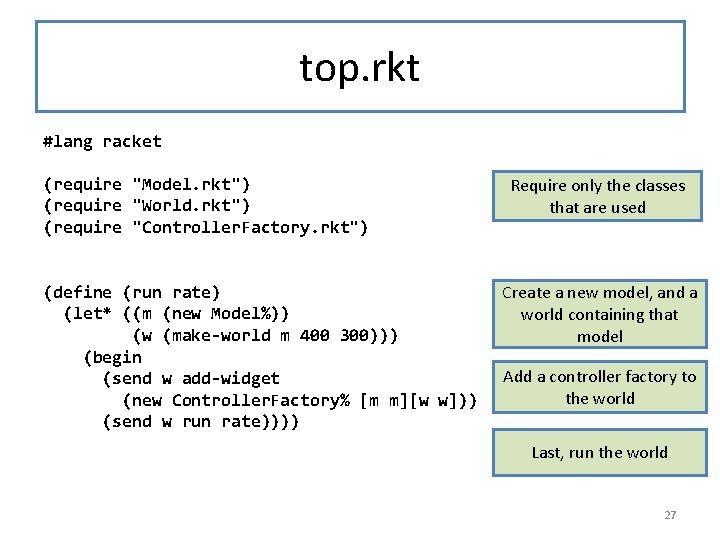top. rkt #lang racket (require "Model. rkt") (require "World. rkt") (require "Controller. Factory. rkt") (define (run rate) (let* ((m (new Model%)) (w (make-world m 400 300))) (begin (send w add-widget (new Controller. Factory% [m m][w w])) (send w run rate)))) Require only the classes that are used Create a new model, and a world containing that model Add a controller factory to the world Last, run the world 27Takeaways from this Lesson • MVC is a widely-used architecture • It is a 3 -tier architecture • It divides the system up into relatively small, easy-to-understand pieces. • 3 interfaces: – world -> controllers – controllers -> model – model -> controllers • 2 publish/subscribe relationships allow controllers to be created dynamically. – world publishes to controllers – model publishes to controllers • Controller -> Model interface is a rudimentary programming language 28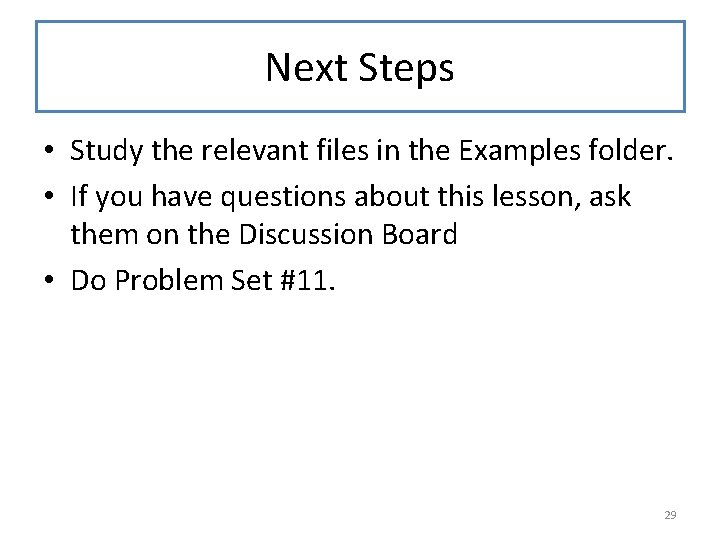Next Steps • Study the relevant files in the Examples folder. • If you have questions about this lesson, ask them on the Discussion Board • Do Problem Set #11. 29